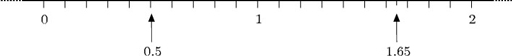Science, Maths & Technology

### Become an OU studentNumbers, units and arithmetic

Start this free course now. Just create an account and sign in. Enrol and complete the course for a free statement of participation or digital badge if available.

# 1.4 Decimals

Quantities can be smaller than one (such as 0.5 kg) or take values between whole numbers (such as a height of 1.65 metres). Numbers smaller than one are expressed as decimals or as fractions. Decimals are often easier to work with (especially when using a calculator). Decimals are explained in this section, and fractions following that (Section 1.7).

Decimals can be indicated on the number line in between whole numbers. 0.5 and 1.65 are indicated on the figure below.Decimals arise when whole numbers are divided by ten, a hundred etc.